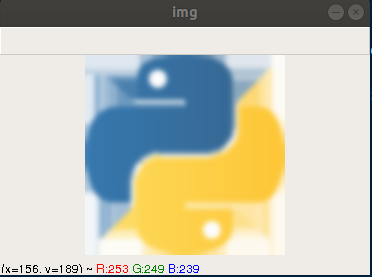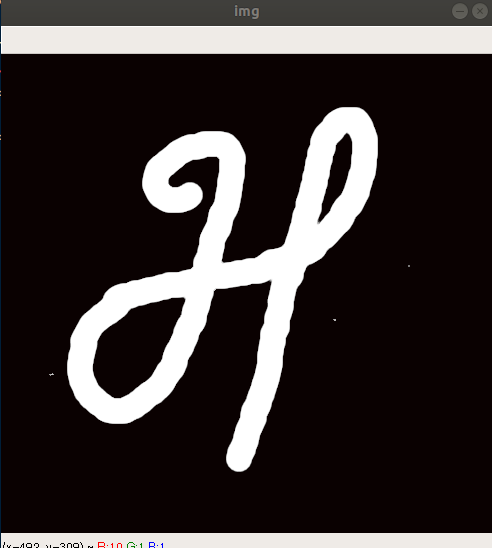## Python Tutorials: Image Filtering Techniques with Python OpenCv

##### Python Tutorials: In this part of Learning Python we Cover Filtering Techniques In Python
Written by |17-Oct-2020 | 0 Comments | 761 Views

Image Blurring techniques:

Image blurring techniques are used for the smoothing of an image. These techniques are used to make the image less sharp and less edgy, so the canny edge detector doesn’t detect every possible small edge in an image. Other than that, it is also used to blur the inappropriate images. For example, we have trained a classifier that classifies the inappropriate images, and we want to that whenever the part in an image came, it gets blurred automatically, so after getting the coordinates of that image, we blurred that part.

This technique is also used to make the image simply because the pixel values are fused into each other, and the sharpness of an image is reduced. There are many types of blurring techniques in OpenCV; we will see all of them one by one:

Averaging:

This technique is used along with the kernel. The kernel window is then iterated all over the image. It takes all the pixel values within a kernel window and replaces the value with the average of all values. This technique normalizes the image and makes it blur. If you want to apply this filter, the following code should be followed:

 import cv2 import NumPy as np img = cv2.imread('logo.png') blur = cv2. blur(img,(5,5)) cv2.imshow("img",blur) cv2.waitKey(0)

The output that is generated as a result is as follows:Figure 1

Now we can see clearly that the image is blurry. So, this is the first method to make the image blurry.

Gaussian Blur:

In this approach, we do not use a standard kernel with an equal filter coefficient. Instead, we use the Gaussian Kernel. In the Gaussian kernel, we should specify the width and height of the kernel. It is to be noted that the kernel width and height should be more than 0 and should be an odd number.

The 3rd parameter it took is a standard deviation that means to what extent the pixel value can deviate along with the X and Y-axis. If only the value of X is given, the system will take equal to Y. The following code is used to blur an image with the help of a Gaussian blur.

 import cv2 import numpy as np img = cv2.imread('logo.png') blur = cv2. GaussianBlur(img,(15,15),0) cv2.imshow("img",blur) cv2.waitKey(0)

In the above code, we have increased the size of the kernel. It is important to write the kernel width and height in odd otherwise system will generate the following error:

 Traceback (most recent call last):   File "/home/lalatoofani/Desktop/Python Module/opencv/1.py", line 6, in     blur = cv2. GaussianBlur(img,(14,14),0) cv2.error: OpenCV(3.4.1) /io/opencv/modules/imgproc/src/smooth.cpp:3186: error: (-215) ksize.width > 0 && ksize.width % 2 == 1 && ksize.height > 0 && ksize.height % 2 == 1 in function createGaussianKernels

The error is generated when we changed the value of kernel to (14,14). It says that there is an error while creating the kernel because the width and height’s modulus with two must generate the value of 1

When we run the above-given code following image is generated:Figure 2

As we have increased the value of the kernel, we can see clearly that the image is more blur than the previous one.

Median Filtering Technique:

In this technique, a kernel is used too. The kernel iterates over the image, and then the central pixel is then converted into the median value of all pixels. This is highly important when removing the small particles. It can reduce the maximum number of noises from the image without affecting the actual image itself.

The salt and pepper noise can be reduced to a great extent, with the help of this filter. Just we saw in the case of an open filter, and we reduce the small particles.

Similarly, we will remove those particles with the help of the median filter. It is to be noted in the case of averaging and the Gaussian filter, and the median value is not the actual value of that pixels; however, in a median filter, the central pixel is from those groups of pixels. This reduces the noise, and similar to the case of the Gaussian filter, the kernel size should be odd and should be positive.

We are going to apply this filter on the following image:Figure 3

In this image, we can see there is a lot of noise. These small particles make noise in an image and so to remove this noise following code is to be run on the image:

 import cv2 import NumPy as np img = cv2.imread('m2.png') blur = cv2.medianBlur(img,5, 5) cv2.imshow("img",blur) cv2.waitKey(0)

We have read an image, and after reading the image, we have applied the median blur on it with the kernel size 5, 5. So after running this code following output is generated:Figure 4

In Figure 4, we can see more than 90% of the noise is removed from the image.

Now the question arises why we use this? So, the answer is when we are performing some tasks on an image, these small particles can be detected as the actual object and can cause an obstruction in recognizing the image. So, it is necessary to remove the noise.

Another essential reason is supposed you have to create a dataset for classifying an image, and you have the images which were taken with the lousy camera, so to make the dataset look clean, we have to remove the noise before feeding into a machine learning algorithm.

Bilateral Blurring Technique:

In all the previous filtering techniques, we have that the edges are not maintained that match the image dilates. However, with the help of bilateral technique, the edges can be preserved, and the other small objects can be blurred. The bilateral method is slower than the other blurring technique because it took more computation than the other.

The code of bilateral blur is as follows:

 import cv2 import NumPy as np img = cv2.imread('ball.jpg') blur = cv2.bilateralFilter(img,9, 75,75) cv2.imshow("img",blur) cv2.waitKey(0)

When to use?

This technique is used when you have to blur the pattern within the actual object; suppose we have an image of wood in which a small pattern can be seen. So, to remove those patterns without changing the edges of that wood, we will use a bilateral filter to filter out those patterns.